# ComputeCpp v1.1.6: Changes to Work-item Mapping Optimization

## 18 November 2019

In ComputeCpp v1.1.6 we have introduced an optimization to the way SYCL work-items map to OpenCL processing elements in order to improve performance in the most common use cases. While this change could have performance implications in some cases, it is straight forward to resolve these. This blog post will start by providing an overview of what has changed and if and how you may need to update your SYCL code. It will then provide further rationale and detail of the change for those who are interested in the implementation details.

## What you need to know

What this change means is that the mapping of a 2 or 3-dimensional nd_range to the underlying OpenCL processing elements has been transposed, such that if you use the SYCL API to translate a 2 or 3-dimensional id into a linear index you will get better performance by default, as this mapping is now more suited to most OpenCL devices. The APIs which perform linearization for you are:

• item::get_linear_id()
• nd_item::get_global_linear_id()
• nd_item::get_local_linear_id()
• accessor::operator[](id)

However, if you manually calculate a linear index (which we do not recommend) then you may see a drop in performance from ComputeCpp v1.1.6, so you will need to reverse the id and range dimensions in your calculation. For example, if you calculated the linear index of a 2-dimensional id using L = id0 + (id1 * r0) you would flip this to L = id1 + (id0 * r1) as we do in the before and after code samples below (Figure 1).

 Before After ``````cgh.parallel_for(range<2>(64, 128), [=](item<2> it) { size_t linearIdx = it.get_id(0) + (it.get_id(1) * it.get_range(0)); outputAcc[linearIdx] = some_func(inputAcc[linearIdx]); });`````` ``````cgh.parallel_for(range<2>(64, 128), [=](item<2> it) { size_t linearIdx = it.get_id(1) + (it.get_id(0) * it.get_range(1)); outputAcc[linearIdx] = some_func(inputAcc[linearIdx]); });``````

Figure 1: Manually linearization, before and after

We recommend that you first measure the performance of your kernels with the ComputeCpp v1.1.6 release and compare that with the ComputeCpp v1.1.5 release in order to determine whether your code is affected by this change. By then measuring the performance before and after any change to linearization you can also ensure it has had the intended effect. If you encounter any issues when applying the change described above, or find a case not covered in this blog post, please feel free to start a thread on our support forum and our team will try to give you the best advice.

## The rationale behind this change

When writing SYCL kernels, particularly for vector processors such as SIMD CPUs and GPUs, one of the most important optimizations ways you can achieve good performance is to ensure that access to global memory is coalesced.

The way to achieve this is to ensure that consecutively executing processing elements access consecutive elements in memory take advantage of vectorized load and store operations. In this scenario the same instruction is performed across a number of consecutive processing elements together, loading and storing many elements of data at once (Figure 2).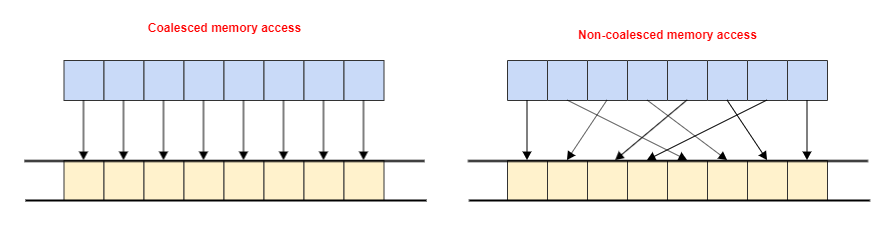Figure 2: Comparison of coalesced and non-coalesced global memory access

If you don't do this then you will likely end up utilizing only part of the vectorized load and store operations, and ultimately require many more load and store operations than you need to. This can result in a pretty significant performance hit, such as that demonstrated in the graph below, taken from a SYCL image convolution kernel (Figure 3).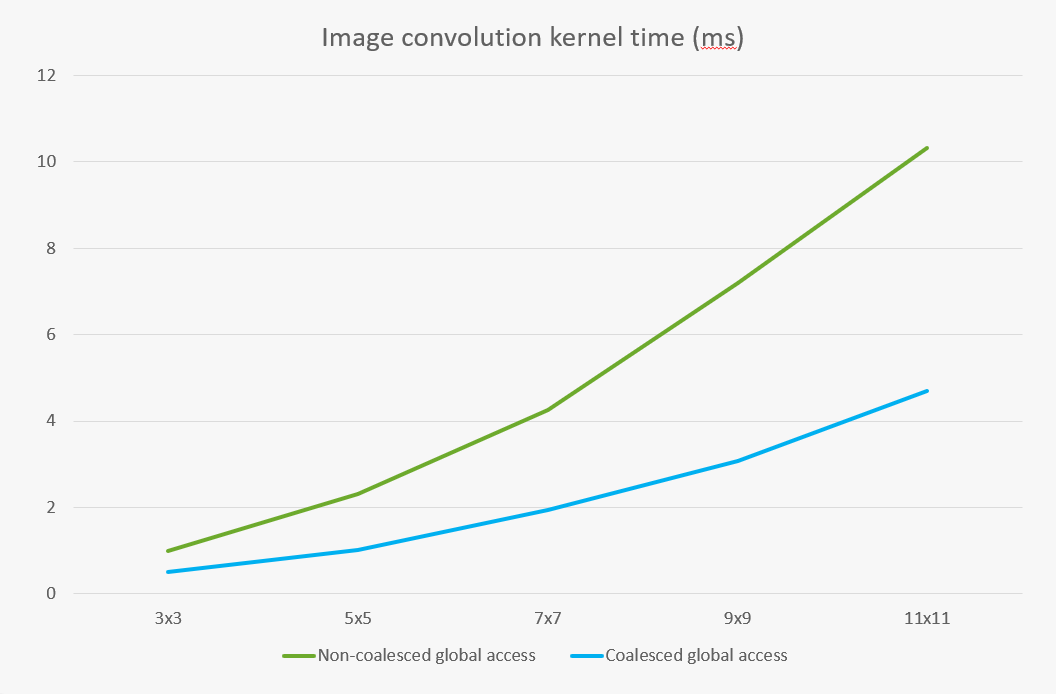Figure 3: Kernel timings comparing coalesced and on-coalesced global memory access

If the nd_range is 1-dimensional this is straightforward because the work-item iteration space is already linear, so the mapping to consecutive processing elements is already coalesced. However, when the nd_range is multi-dimensional, OpenCL doesn't make any guarantee as to how work-items are mapped to consecutive processing elements.

When the nd_range is multi-dimensional things are different. Describing the mapping of indexes in a multi-dimensional nd_range iteration space into a linear iteration space that maps to consecutive processing elements can be described as iteration space linearization, and there are generally two ways in which this can be done by a SYCL implementation. These are often referred to as row-major and column-major, so named because the contiguous indexes once moved to a linear iteration space are along the same row and column in the multi-dimensional space respectively.

In the two examples below we show the nd-range of { 3, 4 } we described earlier, colour-coded with groups of four work-items that are mapped to consecutive processing elements. Figure 4 shows the use of row-major, where the work-items that are consecutive in their mapping to processing elements are in the second dimension. Figure 5 shows the use of column-major, where the work-items that are consecutive in their mapping to processing elements are in the first dimension.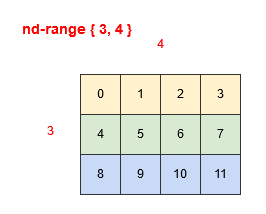Figure 4: Row-major mapping            Figure 5: Column-major mapping

This concept of linearization also applies in how the coordinates of an accessor's two-dimensional data space linearize into a linearized space where consecutive elements are contiguous in memory. This is where it becomes important for performance because depending on whether the linearization of the nd_range iteration space matches the linearization of the accessor data space.

If they do match you get coalesced global memory access, but if they don't match then you get non-coalesced global memory access, which leads to the kind of performance drop we saw earlier.

So the crux of the issue is that the SYCL API performs linearization of multi-dimensional iteration spaces using row-major order, the reason for this is that it maps well to multi-dimensional arrays in C++, which also linearize into contiguous memory following row-major linearization. However, it is most common for OpenCL devices to use column-major linearization for mapping the nd_range iteration space to consecutive processing elements. This meant that the default for the SYCL API, while consistent with C++ was often not the most efficient as seen in Figure 6. However, after the optimization of transposing the mapping of the nd_range iteration space, the linearization of the SYCL API now matches the linearization of the nd_range iteration space to OpenCL processing elements, therefore resulting in coalesced global memory access by default as seen in figure 7.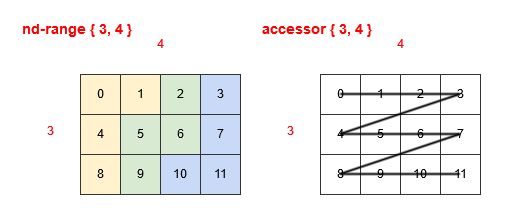Figure 6: Original mapping of work-items to OpenCL processing elements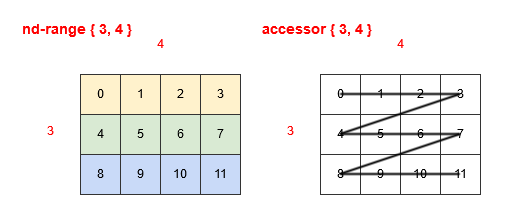Figure 7: New mapping of work-items to OpenCL processing elements